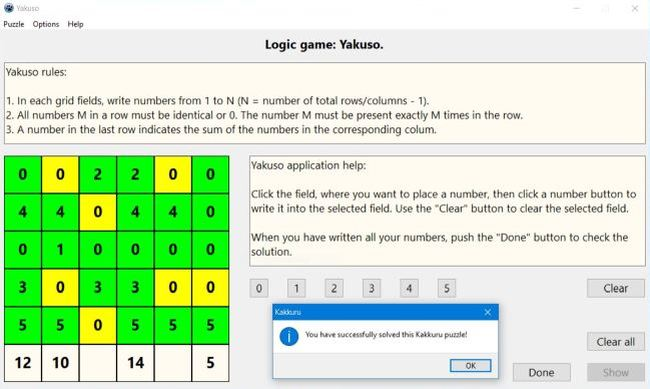# Computing: Free Pascal Programming

 Programming Home Home Contact

## Logic number puzzle: Yakuso (Yakuso).

Description: Puzzle game with numbers to be placed onto a grid. The rules of the game are as follows:
1. In each grid fields, write numbers from 1 to N (N = number of total rows/columns - 1).
2. All numbers M in a row must be identical or 0. The number M must be present exactly M times in the row.
3. A number in the last row indicates the sum of the numbers in the corresponding colum.
The game is a typical logic game: In order to find the correct solution of the puzzle, you have to explore the numbers already writtem and the sums given, then, by logical thinking (concluding from what you know, that a given field must be a given number, or on the contrary, can't be a given number), determine, which fields have to contain which numbers.
The game is played exclusively with the mouse: Click the field, where you want to write a number, then click one of the number buttons to write the corresponding number or "Clear" to remove the number from the actually selected field.

Improvement:: It seems to me that the puzzle actually is to easy to solve. Reviewing the code, how many and which numbers and sums are given by the program, would make the game more interesting for players other than beginners. Addition of difficulty levels would also be a good idea.

Free Pascal features: Changing the color of shapes and the caption of static-texts during execution. Using buttons to insert characters into a given control's caption. Two-dimensional arrays (classic Pascal).

Screenshot: